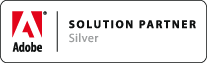Support » Knowledge Base » Documentation Tips and Tricks

# HOW TOs (MathType)

Associated Product: MathTools 2.0

Last change: Mar 13, 2015

### HOW TO Exchange MathType equations with MathTool

• Word document import
• Drag'n'drop from MathType
• `Copy as MathML` and paste into MathType
• Convert placed MathType EPS inline images

### HOW TO Convert inline MathType equations to MathZones

• Perform `Math` > `Enable Math for Current Document`
• Perform `Math` > `MathZone` > `Create from Inlined Equation Image(s)`
• Note: this works on a selection. If no selection exists, the entire document is affected!

### HOW TO Import Word documents with embedded MathType equations

• Perform `Math` > `Enable Math for Current Document`
• Place Word document

### HOW TO Troubleshoot failing conversion of MathType equations

As very first step: check that you've placed the Word document into a Math Enabled document; repeat with a Math Enabled document.

As a second step: check that these failing equations can still be edited in Word!

Otherwise...

We are aware of a few conditions that causes MathTools V2 to fail to convert MathType equations embedded in Word documents:

• MathType equations in Word document, or RTF, were generated by some 3rd party product in an incompatible way
• Word:mac was used to create Word document and MathType equations

Note: these require a full version of MathType from Design Science in Word!

#### Solution 1: Using Word for Windows with MathType

• Open a COPY of the Word/RTF file in Word for Windows
• In Word: perform `MathType` > `Convert Equations` to open the dialog
• In the dialog: check all Equation types
• In the dialog: For 'Convert equations to:' select `MathType equations (OLE objects)`
• Press Convert

This conversion may take a while, depending on the size of the document and its structural complexity. It may even fail with an error, hang, or, crash, or just stop with partial conversion result. In case it does not finish successfully, split the Word document into smaller pieces until conversion succeeds. You may even need to delete inline frames/images, footnotes, tables, and the likes win Word to get the conversion process finish!

Once it finished successfully, save the Word document and repeat the import in InDesign with that Word document. MathTools should now be able to convert the equations.

#### Solution 2: Using Word (Windows or Mac) with MathType

• Open a COPY of the Word/RTF file in Word for Windows
• In Word: perform `MathType` > `Convert Equations` to open the dialog
• In the dialog: check all Equation types
• In the dialog: For 'Convert equations to:' select `Text using MathType translator`
• From the list choose translator `LaTeX 2.0.9 and later`
• Be sure to check both, `Include translator name as comment` and `Include MathType data as comment`
• Press Convert

Once the conversion is finished

• save the document and
• place the resulting file into a Math Enabled InDesign document
• perform `Math` > `MathZone` > `Create from inlined Text-encoded equations`

#### Solution 3: Using Word (Windows or Mac) with MathType

This solution exports all the equations to external EPS files which can be placed in InDesign and converted to MathZones as a subsequent step. This most likely makes sense for more complex equations.

• Open a COPY of the Word/RTF file in Word for Windows
• In Word: perform `MathType` > `Export Equations` to open the dialog
• In the dialog: Choose file type `Encapsulated PostScript/PICT` (for MAC) or `Windows Meta File` (for Windows)

Once all the equation images are exported, you can start to place them into the respective places in the InDesign text story. Follow HOW TO Convert inline MathType equations to MathZones (above) to convert these equations

#### Solution 4: Copy & Paste equations from the Word document to InDesign

This most likely makes sense for more complex equations.

• Open the Word/RTF document in Word
• Copy the equation in Word
• Paste the equation into the InDesign story using `Paste as MathZone`movemen is an Adobe Solution Partner.